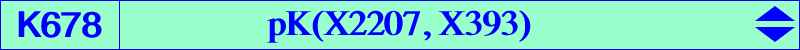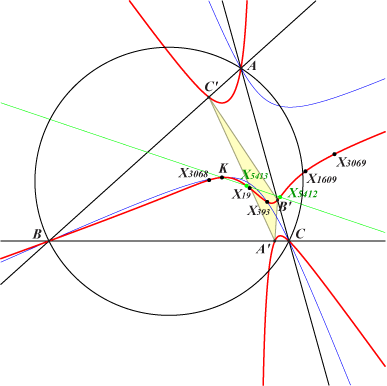∑ SA^2 (c^2 y - b^2 z) x^2 = 0 or ∑ a^2 (SB^2 y - SC^2 z) y z = 0X(6), X(19), X(393), X(1609), X(3068), X(3069), X(5412), X(5413) vertices of the cevian triangle of X(393) vertices of the anticevian triangle of X(19)K678 is the transform of the Orthocubic K006 under the mapping f : u:v:w -> (SB v - SC w) / (c^2v^2 - b^2w^2) : : . See details in the page K095 and also Q053. K678 is also the barycentric product of K006 by X(4). It is therefore anharmonically equivalent to the Orthocubic K006. The isogonal transform of K678 is K857. Note that the pole X(2207) of K678 is the barycentric product of the pivot X(393) and the Lemoine point K whose polar conic is the circum-conic passing through G, K, X(393) and many other points. The square roots of the pole X(2207) are X(19) and the vertices of its anticevian triangle. In fact, the restriction of f to K006 is the barycentric product of M and the orthocenter H. In other words, for any M on K006, the point f(M) = H x M lies on K678. Note that, in this case, f is a birational transformation. The following table gives the correspondence of M and f(M).M X(1) X(3) X(4) X(46) X(90) X(155) X(254) X(371) X(372) X(485) X(486) X(487) X(488) f(M) X(19) X(6) X(393) ? ? X(1609) ? X(5413) X(5412) ? ? X(3069) X(3068)Remark : two isogonal conjugate points M, N on K006 (hence collinear with H) give two isoconjugate points f(M), f(N) collinear with X(393). Furthermore, the lines M, f(M) and N, f(N) intersect on the Brocard axis OK.